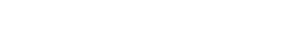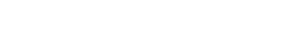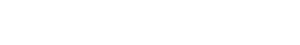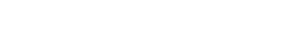4.2.2 Transverse & Longitudinal Waves

Transverse & Longitudinal Waves

Transverse Waves

• Transverse waves are defined as follows:

Waves that oscillate perpendicular to the direction of motion and energy transfer

• Light waves are an example of transverse waves
• Transverse waves do not need particles to propagate, and so they can travel through a vacuum

Longitudinal Waves

• Longitudinal waves are defined as follows:

Waves that oscillate parallel to the direction of motion and energy transfer

• Sound waves are an example of longitudinal waves
• Longitudinal waves need particles to propagate, and so they cannot travel through a vacuum
• As a longitudinal wave propagates, areas of low and high pressure can be observed:
• A rarefaction is an area of low pressure, with the particles being further apart from each other
• A compression is an area of high pressure, with the particles being closer to each other

Worked Example

The diagram below represents a transverse wave at time t = 0. The direction of motion of the wave is shown. Point P is a point on the wave.

State in which direction point P will move immediately after the time shown.

Step 1: Recall that transverse waves oscillate perpendicular to the direction of motion

• This transverse wave travels from right to left
• Oscillations will either be up or down
• Hence point P will either move up or down

Step 2: Appreciate that, since the wave is moving from right to left, a crest (i.e. a point of maximum displacement above the equilibrium position) will be approaching point P immediately after the time shown

• Point P will be moving upwards

Transverse & Longitudinal Graphs

• Two different types of graphs can be used to represent a travelling wave:
• Displacement-distance graphs, with displacement x (m) on the y-axis and distance d (m) on the x-axis
• Displacement-time graphs, with displacement x (m) on the y-axis and time t (s) on the x-axis
• Both transverse and longitudinal waves can be shown on these graphs

Displacement-Distance Graphs

• A displacement-distance graph is also known as a wave profile
• It represents the displacement of many particles on the wave at a fixed instant in time (e.g. t = 0)
• A displacement-distance graph directly provides:
• The amplitude A of the wave
• The wavelength λ of the wave

• In the displacement-distance graph of a transverse wave moving in the horizontal direction:
• Particles with positive displacement are those moving up
• Particles with negative displacement are those moving down
• In the displacement-distance graph of a longitudinal wave moving in the horizontal direction:
• Particles with positive displacement are those moving to the right
• Particles with negative displacement are those moving to the left

Displacement-Time Graphs

• A displacement-time graphs represents the variation of the displacement of one particle with time
• A displacement-time graph directly provides:
• The amplitude A of the wave
• The period T of the wave

Worked Example

Below is the displacement-time graph for a light wave travelling at 3 × 108 m s–1.

Determine:

• The period of the wave in seconds (s)
• The wavelength of the wave in metres (m)

Step 1: Determine the period T directly from the displacement-time graph

• Recall that period is defined as the time taken for one complete oscillation
• Note that you must convert the period from milliseconds (ms) into seconds (s)

T = 2 × 10–3 s

Step 2: Write down the relationship between frequency f and period TStep 3: Substitute the value of the period determined in Step 1 into the above equationf = 500 Hz

Step 4: Write down the wave equation

c = fλ

Step 5: Rearrange the above equation to calculate the wavelength λStep 6: Substitute the velocity c = 3 × 108 m s–1 and the frequency f calculated in Step 3λ = 6 × 105 m

Exam Tip

When approaching a question, pay attention to the label on the x-axis of the graph.

• The distance between two adjacent crests (or troughs) on a displacement-distance graph is equal to the wavelength λ of the wave
• The distance between two adjacent crests (or troughs) on a displacement-time graph is equal to the period T of the wave

Remember to look at the units of measure on both axes of the graph, and convert units if needed.

CloseClose